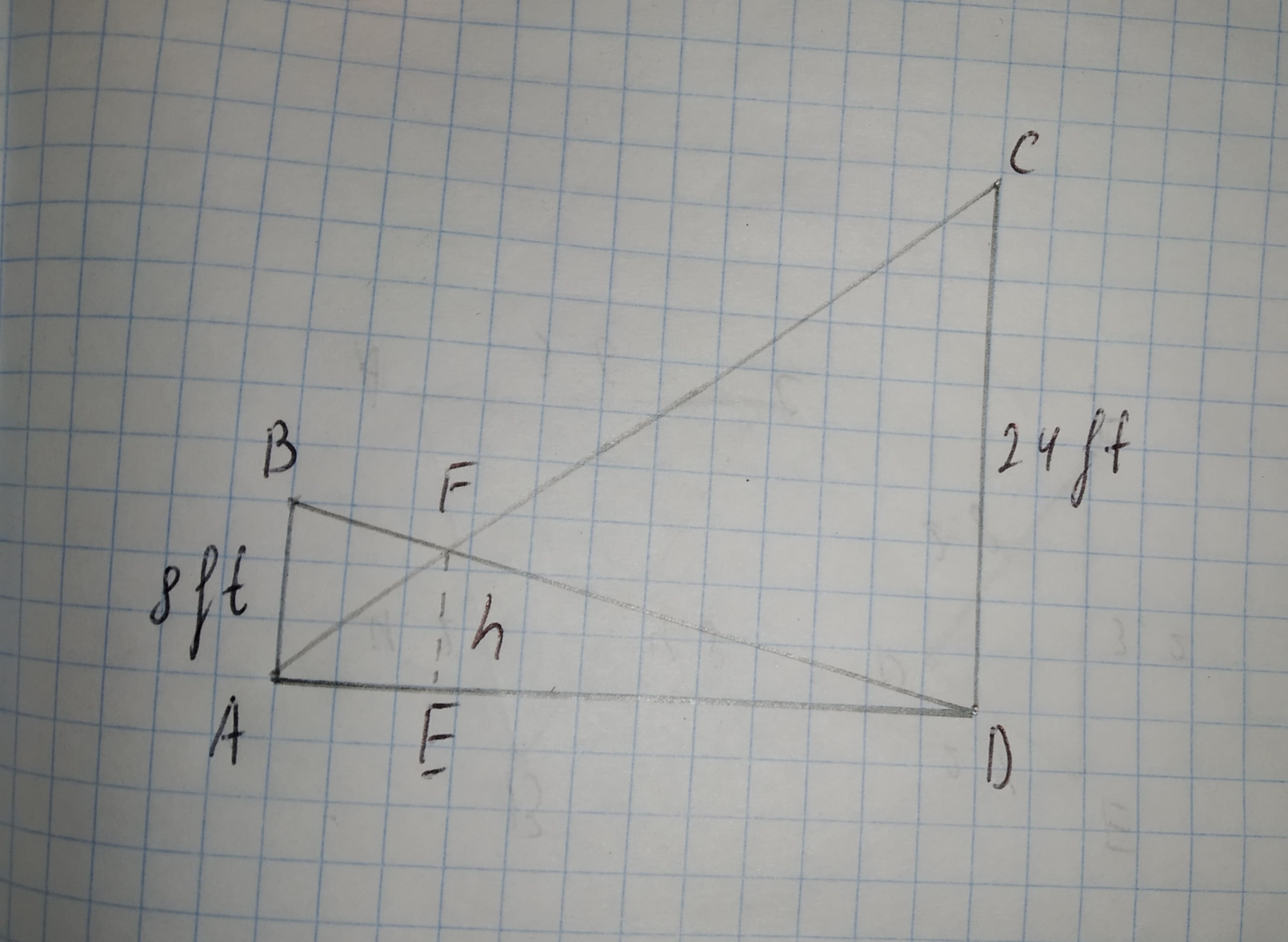# Finding a Lenght Two vertical poles, one 8 ft tall and the other 24 ft tall, have ropes stretched from the top of each to the base of the other (see the figure). How high above the ground is the point where the ropes cross? [Hint: Use similarity]Dottie Parra 2021-01-19 Answered
Finding a Lenght Two vertical poles, one 8 ft tall and the other 24 ft tall, have ropes stretched from the top of each to the base of the other (see the figure). How high above the ground is the point where the ropes cross? [Hint: Use similarity]
You can still ask an expert for help

• Questions are typically answered in as fast as 30 minutes

Solve your problem for the price of one coffee

• Math expert for every subject
• Pay only if we can solve itSabadisO

Given:
One pole is 8 ft tall and other pole is 24 ft tall.
Both have ropes stretched from the top of each to the base of the other.Figure(1)
Approach:
If all three angles of a triangle are equal to corresponding angles of the other triangle, then both the triangles are similar and the rule is referred to as AAA rule.
Calculation:
By AAA rule, triangle AEF is similar to triangle ADC.
So,
$\frac{AE}{h}=\frac{AE+ED}{DC}$
$=\frac{AE+ED}{24}$...(1)
By AAA Rule, triangle BAD is similar to triangle FED.
So,
$\frac{ED}{h}=\frac{AE+ED}{A}B$
$=\frac{AE+ED}{8}$
$AE+ED=\frac{8ED}{h}$...(2)
Substitute value AE+ED from equation (2) in equation (1).
$\frac{AE}{h}=\frac{8ED}{h}/24=\frac{8ED}{24h}$
$AE=\frac{ED}{3}$
Substitute $\frac{ED}{3}$ for AE in Equation (2).
$\frac{AE}{3}+ED=\frac{8ED}{h}$
$\frac{4ED}{3}=\frac{8ED}{h}$
$\frac{4}{3}=\frac{8}{h}$
$h=\frac{3×8}{4}$
=6
Therefore, the height of the point above the ground where the ropes cross is 6 ft.